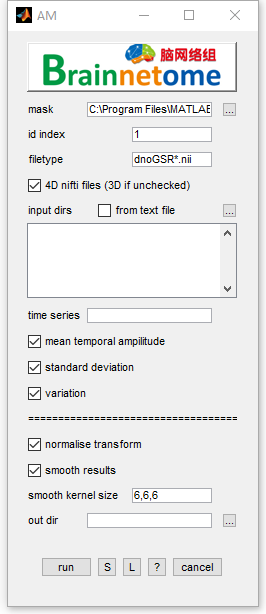AM¶

AM is calculated as the average amplitude and the standard deviation of the mean-subtracted time series. The AM represents the strength of time series’ temporal fluctuation, which is similar to ALFF/fALFF.• id index: identifier to find unique string for each subject
• filetype: files in the filetype will be searched in input directories.
• 4D nifti files: if the input data is 4D, check this item. Otherwise uncheck.
• input dirs: directories can be input either using a `*.txt` file or spm select window.
• mean temporal ampilitude: calculate absolute value of detrended and demeaned time series.
• standard deviation: calculate standard deviation of time series
• variation: calculate variation of time series
• normalize transform: in output file, a suffix of _m means the output is divided by mean intensity in the mask; a suffix of _z means the output is subtracted by mean intensity and divided by standard deviation.
• out dir: output directory for saving results.
• Buttons:
• S: Save parameters of the current panel to a `*.mat` file. The `*.mat` can be further loaded for the panel or be used in a script processing.
• L: Load parameters from `*.mat` for the current panel.
• ?: Help information.
• Reference:
1. Liu Y, Yu C, Zhang X, Liu J, Duan Y, Alexander-Bloch AF, et al. Impaired long distance functional connectivity and weighted network architecture in Alzheimer’s disease. Cereb Cortex 2014; 24(6): 1422-35.